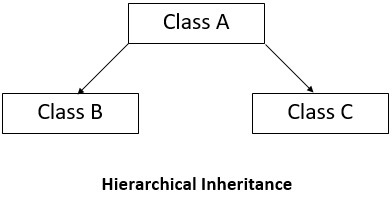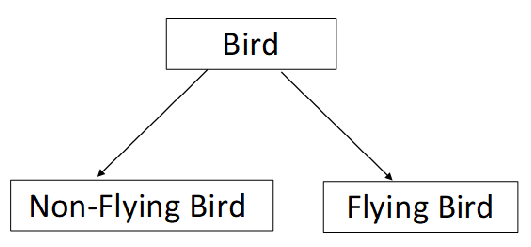×

Search anything:

# Hybrid Inheritance in C++

#### C++ Software Engineering inheritance hybrid inheritanceFREE BOOK -> Problems for the day before your Coding Interview (on Amazon)

Reading time: 20 minutes | coding time: 2 minutes

Hybrid inheritance is done when we have to mix different types of inheritance within a single program, for example, mixing single inheritance with multiple inheritance or multiple inheritance within a single program.• In the above diagram, Class B and Class C are derived from the base class A.

• Example-In the above figure, a hierarchical classification of birds is shown. Here there are two derived classes 'Non-Flying Bird' and 'Flying Bird' accesses the members of one base class 'Bird'.

### Example Code

``````#include<iostream>
#include<conio>
using namespace std;
class arithmetic
{
protected:
int num1, num2;
public:
void getdata()
{
cout<<"\nEnter the first number: ";
cin>>num1;
cout<<"\nEnter the second number: ";
cin>>num2;
}
};
class plus:public arithmetic
{
protected:
int sum;
public:
{
sum=num1+num2;
}
};
class minus
{
protected:
int n1,n2,diff;
public:
void sub()
{
cout<<"\nFor Subtraction:";
cout<<"\nEnter the first number: ";
cin>>n1;
cout<<"\nEnter the second number: ";
cin>>n2;
diff=n1-n2;
}
};
class result:public plus, public minus
{
public:
void display()
{
cout<<"\nSum of "<<num1<<" and "<<num2<<"= "<<sum;
cout<<"\nDifference of "<<n1<<" and "<<n2<<"= "<<diff;
}
};
int  main()
{
result z;
z.getdata();
z.sub();
z.display();
return 0;
}
``````

Output:

``````Enter the first number:10
Enter the second number:5
``````

For Subtraction:

``````Enter the first number:10
Enter the second number:5

Sum of 10 and 5= 15
Difference of 10 and 5= 5
``````